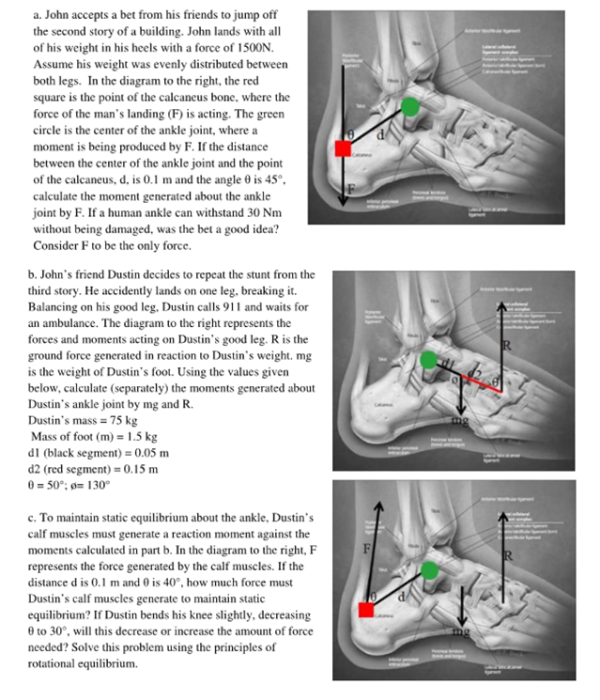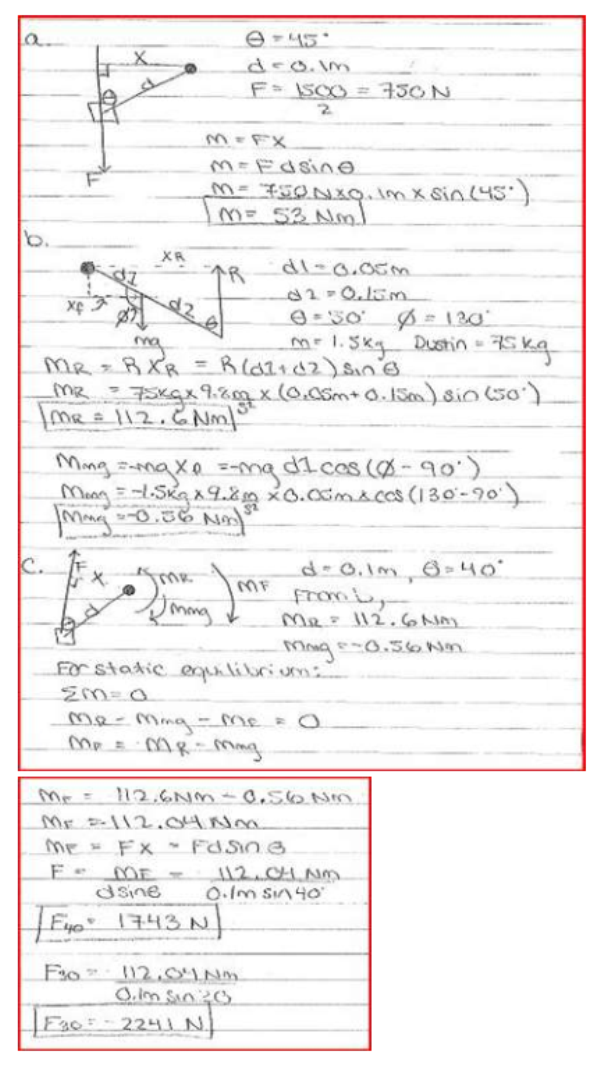John accepts a bet from his friends to jump off the second story of a building. John lands with all of his weight in his heels with a force of 1500 N. Assume his weight was evenly distributed between both legs. In the diagram to the right, the red square is the point of the calcaneus bone, where the force of the man's landing is acting. The green circle is the center of the ankle joint, where a moment is being produced by F. If the distance between the center of the ankle joint and the piont of the calcaneus, d, is 0.1 m and the angle theta is 45 degrees, calculate the moment generated about the ankle joint by F. If a human ankle can withstand 30 Nm without being damaged, was the bet a good idea? Consider F to be the only force.John accepts a bet from his friends to jump off the second story of a building. John lands with all of his weight in his heels with a force of 1500 N. Assume his weight was evenly distributed between both legs. In the diagram to the right, the red square is the point of the calcaneus bone, where the force of the man's landing is acting. The green circle is the center of the ankle joint, where a moment is being produced by F. If the distance between the center of the ankle joint and the piont of the calcaneus, d, is 0.1 m and the angle theta is 45 degrees, calculate the moment generated about the ankle joint by F. If a human ankle can withstand 30 Nm without being damaged, was the bet a good idea? Consider F to be the only force.

biomechanics page 1 biomechanics biomechanics biomechanics biomechanics biomechanics biomechanics biomechanics biomechanics page 2